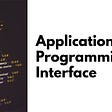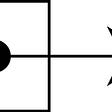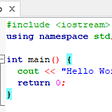# Dynamic Memory Allocation in C

1. Stack: A stack is a area of computer’s memory which stores variables created by a function. In stack, variables are declared, stored and initialized during runtime and destroyed after program execution.
2. Heap: The heap is used by programming languages to store global variables. It supports Dynamic memory allocation. Heap does not destroy data itself.

# Dynamic Memory Allocation(DMA)

## Example

`int *x;x = (int*)malloc(150 * sizeof(int)); `

## Syntax

`void *calloc(items, size);`

## Example

`int *x;x = (float*) calloc(150, sizeof(float));`

## Syntax

`void* realloc(pointer, new-size)`

## Example

`int *x;x = (int*)malloc(150 * sizeof(int));x = (int*)realloc(x,300);`

## Syntax

`free(ptr);`

## Example

`int *x;x = (int*)malloc(150 * sizeof(int));free(x);`

# Dynamic Memory Allocation Example

`#include <stdio.h>#include <stdlib.h>void main(){    int i, n;    int *x;    printf("Enter total number of elements: ");    scanf("%d", &n);    x = (int*) calloc(n,sizeof(int));         if(x == NULL)     {        printf("Not enough space.");    }    for(i = 0; i < n; i++)    {       scanf("%d", x+i);     }    for(i = 1; i < n; i++)    {       if(*x < *(x+i))       {            *x = *(x+i);        }    }    printf("Greatest number is %d", *x);    free(x);}`

--

--

--

## More from crysp

Hola! They call me Hacker

Love podcasts or audiobooks? Learn on the go with our new app.

## Why Should You Learn Rust?## The Hardest Part is Just to Start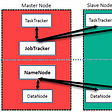## How CSS & HTML Interact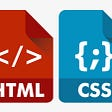## Left rotation of an array using vectors in C++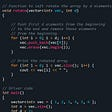## The tools, they are a-changing## CS371p Fall 2021: Week 2## Tutorial No.2 for Plato Farm’s newcomers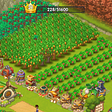## crysp

Hola! They call me Hacker

## Application Programming Interface